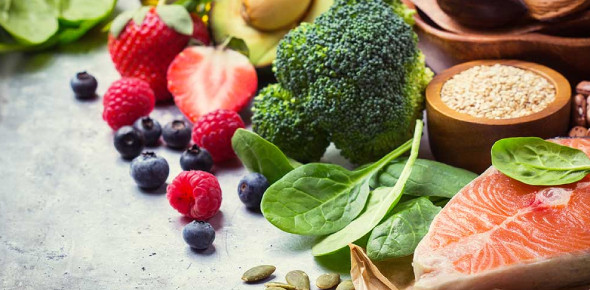# Minerals In The Body Quiz

26 Questions | Total Attempts: 331SettingsThe body needs energy, which is gotten from minerals, whereas some it can produce naturally there are others we need to supplement by eating in foods or fruits. Do you know just the right amounts that are healthy for the body? Take up the test below and see just how much you know about minerals in our bodies.

• 1.
Calcium is a:
• A.

Intracellular mineral

• B.

Extracellular mineral

• C.

Can be both

• 2.
There is approximately __________ kilograms of calcium in the average adult male
• A.

1 Kilogram

• B.

1.2 Kilogram

• C.

1.4 Kilogram

• D.

.800 Kilogram

• 3.
_____________ grams of calcium is exchanged each day between bones & blood.
• A.

400

• B.

500

• C.

600

• D.

700

• E.

800

• 4.
Which mineral maintains the pH of body fluids including urine?
• A.

Calcium

• B.

Sodium

• C.

Phosphate

• D.

Chloride

• 5.
Which mineral is a cofactor to salivary amylase, pancreatic enzymes & phosphorylase B in liver
• A.

Calcium

• B.

Sodium

• C.

Phosphate

• D.

Chloride

• 6.
Aldosterone is associated with which mineral?
• A.

Calcium

• B.

Sodium

• C.

Phosphate

• D.

Chloride

• E.

Potassium

• 7.
Which mineral is the major intracellular cation?
• A.

Calcium

• B.

Sodium

• C.

Potassium

• D.

Phosphate

• E.

Magnesium

• 8.
Which mineral would be used therapeutically for Arrhythmia & Tachycardia?
• A.

Sodium

• B.

Calcium

• C.

Magnesium

• D.

Potassium

• 9.
Which mineral is an essential component of chlorophyll molecule?
• A.

Calcium

• B.

Sodium

• C.

Magnesium

• D.

Potassium

• 10.
Which minerals should you NOT give in renal disease? Mark all that apply...
• A.

Sodium

• B.

Magnesium

• C.

Potassium

• D.

Phosphoros

• E.

Calcium

• 11.
Which mineral requires Vitamin D and PTH to be absorbed?
• A.

Sodium

• B.

Calcium

• C.

Potassium

• D.

Magnesium

• 12.
When Serum Calcium is low, PTH is secreted. Which is NOT a effect of PTH?
• A.

Increases Bone deposition of calcium

• B.

Increases calcium release from bone

• C.

Increases activation of Vitamin D

• D.

Increases calcium reabsorption from urine by kidneys

• 13.
Canned fish is a good source of which mineral?
• A.

Sodium

• B.

Calcium

• C.

Magnesium

• D.

Potassium

• 14.
The hormones adlosterone, oestrogen, progesterone cortisone all increase the loss of which mineral?
• A.

Sodium

• B.

Potassium

• C.

Calcium

• D.

Phosphorus

• 15.
Phytates & oxalates decrease the absorption of which minerals?
• A.

Sodium

• B.

Magnesium

• C.

Calcium

• D.

Potassium

• 16.
Which mineral is a cofactor and activates Thiamin, B6, Niacin?
• A.

Sodium

• B.

Phosphorus

• C.

Calcium

• D.

Magnesium

• 17.
Calcitriol and PTH do what to calcium...
• A.

Increases excretion

• B.

Decreases excretion

• C.

Neither

• 18.
Calcium supplements also decrease the absorption of which mineral?
• A.

Sodium

• B.

Iron

• C.

Magnesium

• D.

Calcium

• 19.
Absorption of calcium occurs best in which environment?
• A.

Acidic

• B.

Alkalising

• C.

Either

• 20.
Excessive intakes of lysine increase the excretion of which mineral?
• A.

Magnesium

• B.

Calcium

• C.

Phosphorus

• D.

Sodium

• 21.
Disfunction of which glands can cause a calcium imbalance?
• A.

Para thyroid

• B.

Thyroid

• C.

Both

• D.

Neither

• 22.
High doses of which hormone - as seen in cushings disease increases renal excretion of Calcium?
• A.

Mineralocorticords

• B.

Corticosteroids

• C.

Androgens

• 23.
Calcium competes with which mineral in the Small Intestine?
• A.

Zinc, manganese, copper, iron

• B.

Zinc, magnesium, copper, iron

• C.

Zinc, manganese, copper, sodium

• D.

Zinc, magnesium, sodium, copper

• 24.
Which mineral protects against heavy & toxic metals?
• A.

Magnesium

• B.

Sodium

• C.

Calcium

• D.

Phosphorus

• 25.
Which mineral is bound to the plasma protein albumin?
• A.

Magnesium

• B.

Zinc

• C.

Calcium

• D.

Chloride

Related TopicsBack to top